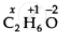CBSE (Science) Class 11CBSE
Share

# What Are the Oxidation Numbers of the Underlined Elements in Each of the Following and How Do You Rationalise Your Results?Ch3ch2oh - CBSE (Science) Class 11 - Chemistry

ConceptOxidation Number - Introduction

#### Question

What are the oxidation numbers of the underlined elements in each of the following and how do you rationalise your results?

CH3CH2OH

#### Solution 1

By conventional method O.N of C  in CH_3CH_2OH =or 2x + 6(+1) + 1(-2) = 0 or x = -2

#### Solution 2

CH3CH2OH

2(x) + 6(+1) + 1(-2) = 0 or 2x + 4 = 0

or x = -2

Hence, the O.N. of C is –2.

Is there an error in this question or solution?

#### APPEARS IN

Solution What Are the Oxidation Numbers of the Underlined Elements in Each of the Following and How Do You Rationalise Your Results?Ch3ch2oh Concept: Oxidation Number - Introduction.
S